• 如果矩阵是对角矩阵，那么是元素的-1/2。 如果是一般矩阵， 由于矩阵开方后，它的特征值会开方，但是特征向量不变。

如果矩阵是对角矩阵，那么是元素的-1/2。
如果是一般矩阵，由于矩阵开方后，它的特征值会开方，但是特征向量不变。展开全文• 因此，此提交帮助人们使用矩阵的双对角化，A = Q * B * P'，其中 A 是非平方的复数或实数，B 是双对角的实数，Q 和 P 是酉或正交的。 最初的 Lapack 接口例程来自 Tim Toolan 在“文件交换”中的工作。 此代码可...matlab
• sexpm(A) 计算方阵 A 的矩阵指数。... 该算法的详细信息在Stefan Guettel 和 Yuji Nakatsukasa，矩阵指数的缩放和平方对角 Pade 近似，pdf，SIAM 矩阵分析和应用杂志，卷。 37(1)，第 145-170 页，2016 年。matlab
• 　最终得到了S和一个以特征值为对角线的对角矩阵的乘积，这个对角矩阵就是特征值矩阵，用Λ表示： 　没有人关心线性相关的特征向量，上式有意义的前提是S由n个线性无关的特征向量组成，这意味着S可逆，等式两侧...

特征值矩阵

假设A有n个线性无关的特征向量x1,x2……xn，这些特征向量按列组成矩阵S，S称为特征向量矩阵。来看一下A乘以S会得到什么：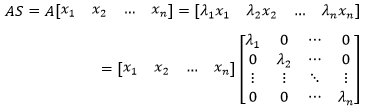最终得到了S和一个以特征值为对角线的对角矩阵的乘积，这个对角矩阵就是特征值矩阵，用Λ表示：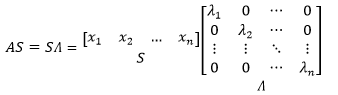没有人关心线性相关的特征向量，上式有意义的前提是S由n个线性无关的特征向量组成，这意味着S可逆，等式两侧可以同时左乘S-1：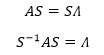AS=SΛ和S-1AS=Λ就是对角化的两种方法。需要注意的是，并非所有矩阵A都存在n个线性无关的特征向量，这类矩阵不能对角化。

矩阵对角化还有另一种表达：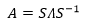我们已经知道了矩阵的LU分解，A=LU；格拉姆-施密特正交化，A=QR；现在有多了一种对角化分解，A=SΛS-1

矩阵的方幂

如果A存在特征值和特征向量，即Ax = λx，那么A2的特征值和特征向量是什么？

这在上一章的示例中出现过，将Ax = λx的等式两侧同时左乘A就可以表示A的特征向量：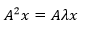由于λ是标量，所以可以把λ单独提出来：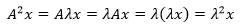现在可以得出结论了，A2的特征向量不变，特征值变成了λ2

可以用同样的方式看看A2的对角化：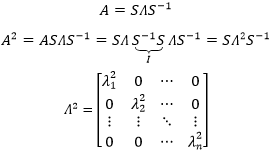按照这个思路可以继续计算Ak的对角化，Ak的特征向量不变，Ak的特征值矩阵是A的特征值矩阵的k次方：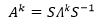根据上式，如果k→∞，在所有特征值|λi|<0时，Ak→0，当然，前提是A有n个线性无关的特征向量。

对角化的前提

对角化的前提是A存在n个线性无关的特征向量，问题是怎样判断A存在n个线性无关的特征向量？一个判断方法是：当A的所有特征组互不相同时，A必然存在n个线性无关的特征向量；如果存在重复的特征值就不好说了，需要另行判断。

n阶单位矩阵的所有特征值都是1，但是它仍然有n个线性无关的特征向量，因此单位矩阵可以对角化：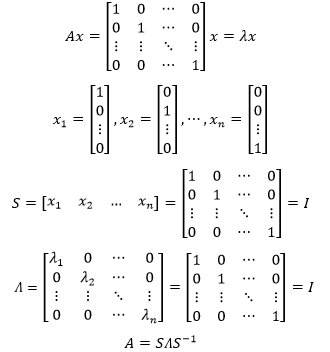再来看三角矩阵。三角矩阵A的各列是线性无关的，意味着它有唯一解，没有n个线性无关的特征向量，比如下面这个：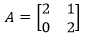先计算A的特征值：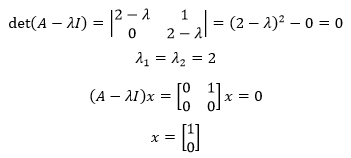作为2×2矩阵，A只有一个特征向量，它无法完成对角化。

使用对角化

给定一个向量u0和一个能够对角化的矩阵A，如果uk+1=Auk，那么u100 = ?

可以简单的向后推导一下：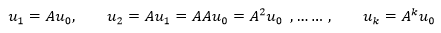现在可以得到结论，u100=A100 u0，问题是如何求得A100？

A有n个线性无关的特征向量x1,x2,……,xn，这意味着u0可以看成这些特征向量的线性组合：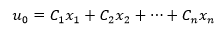以单位矩阵为例，假设A是3×3的单位矩阵，则A的三个特征向量是：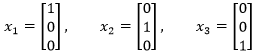这三个特征向量可以通过线性组合成为任意的三维向量。

现在可以将Au0写成下面的形式：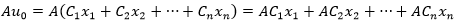由于Ci是标量，所以可以将Ci写到前面：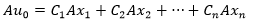x1,x2,……,xn都是A的特征向量，它们以特征值为媒介和A存在关联，Axi = λixi，因此：等式两侧同时左乘A：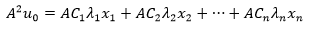同样，可以把比标量Ciλi放到前面：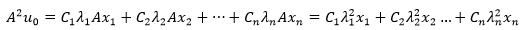无论等式两侧再左乘几个A都将得到类似的结果，因此：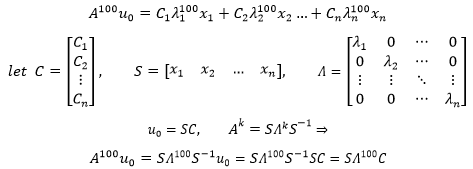这就是最终的答案，如果真要计算A100 u0，可以先把u0展开成特征向量的线性组合，求出具体的C值，在使用SΛ100C求解。

综合示例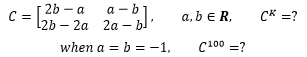a,b都是0的时候没什么可算的，主要看ab≠0的情况。C看起来比较别扭，还是用A来说话。先来看一下特征值：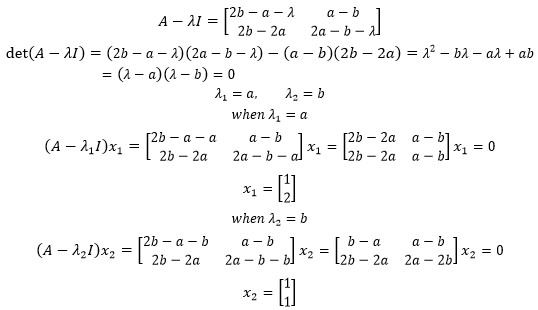特征值矩阵和特征向量矩阵是：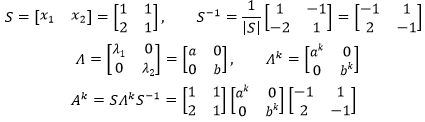当a=b=-1时：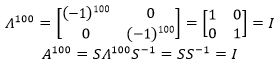作者：我是8位的

本文以学习、研究和分享为主，如需转载，请联系本人，标明作者和出处，非商业用途！

扫描二维码关注公众号“我是8位的”展开全文矩阵的对角化 特征值矩阵 特征向量矩阵
• 为这节矩阵对角化做准备 核心公式 S-1AS=Λ （其中S为特征向量矩阵还有S的逆必须存在） 假设A有n个线性无关特征向量，按列组成特征向量矩阵S 算一下AS=A[x1,x2,…xn]=[ λ1x1, λ2x2,… λnxn]=SΛ= 只要是n个...

上一节讲了Ax= λx 我们可以解出特征值和特征向量 那我们解出来之后要干什么？-- 为这节矩阵对角化做准备

本节 核心公式 S-1AS=Λ

（其中S为特征向量矩阵还有S的逆必须存在）

假设A有n个线性无关特征向量，按列组成特征向量矩阵S
算一下AS=A[x1,x2,…xn]=[ λ1x1, λ2x2,… λnxn]=SΛ=下图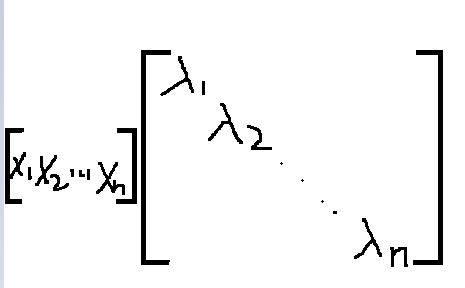只要是n个线性无关特征向量就好

现在我们得到AS=SΛ，又因为S可逆

所以
S-1AS=Λ;
（为什么强调S可逆呢，因为是存在一部分矩阵，特征向量不够n个。）
另一种表达方式
A=SΛS-1
新的分解方式！（LU，QRinGram-Schmidt）也是最重要的！
分解为一个矩阵×对角矩阵×第一个矩阵的逆

我们来使用它

下面考虑一下A2的特征向量和特征值
A2x=λAx=λ2x
发现A2的特征值是λ2
特征向量还是x
我们可以采用另一种方法求解
A2=SΛS-1S-1ΛS=SΛ2S-1
S不变，Λ变为平方
说明特征向量不变，λ变为平方
当然我们可以得到
Ak=SΛkS-1
换句话说，特征值和特征向量提供了理解矩阵幂的一个好方法
如果将矩阵100次方，用LU分解没法做，但是用SVD很简单
特征值是计算矩阵幂的好方法。
例如：什么条件下矩阵的幂趋于0，这样的矩阵称为稳定的
Ak→0 as k→∞
显然取决于Λk
所以需要|λi|<1
再强调一下，所有以上都有一个前提条件，存在n个线性无关的特征向量，如果没有，就不能对角化 。
下面讲一个定理
A必然存在n个线性无关的特征向量（而且可对角化），当特征值互不相同时。
如果特征值重复，可能但不一定存在n个线性无关的特征向量。这不是个完全否定的，比如10×10单位矩阵，特征值都是1，但是任何向量都可以是n维非零向量都是单位矩阵特征向量。
（Ax=λx→A为单位矩阵，x=λx→λ=1→x非零）
单位矩阵本来就是对角矩阵，那么Λ=A

再让我们讲一个三角矩阵的例子

A=[2 1;0 2]
这可能是个麻烦
来分析它，首先要计算特征值和特征向量
|A-λI|=0=|2-λ 1;0 2-λ|=(2-λ)2-1=0
λ1=λ2=2;
再求特征向量，就是这个矩阵的零空间
带回特征值A-2I=[0 1;0 0]
零空间是一维的，代数重度是2，说明重根。
So特征向量仅有x1=[1;0]，这也说明了A 无 法 对 角 化 \color{red}{无法对角化}

差分方程

我不打算深入讨论特征值出现重根的情况。
现在还是围绕之前那个方程（前提是n个无关的特征向量，可以进行对角化）
每个特征值对应一个特征向量，每个特征向量线性无关
下面让我们求解这个方程 U k+1=A Uk
题目给出Uo
Uk=AkUo
这就到了差分方程，一阶差分方程
如何算出U100
如何真正求解U100
把Uo作为一个线性组合，然后乘以A
Uo=c1x1+c2x2+…+cnxn
AUo==c1λ1x1+c2x2λ2+…cnλnxn;

A 100 Uo=c1λ1 100 x1+c2λ2 100 x2+…cn λn 100 xn;
100 Sc
因此，如果真需要计算A的100次方乘以Uo
可以先把Uo展开成特征向量的线性组合
这是我们接下来的公式，下面举个斐波那契数列的例子

展开全文• 以主对角线为对称矩阵MATLAB代码使用Jacobi特征值方法进行奇异值分解 说明 matlab的此存储库中暗含了奇异值分解。 给定的算法可以将输入作为图像文件和简单的矩阵格式。 与内建的matlab函数[U，S，V] = svd（A）的...
• 特征向量对角化一个矩阵：3、假设n×nn\times n矩阵有nn个线性无关的特征向量，如果这些向量是矩阵SS的列，那么S−1ASS^{-1}AS是一个对角矩阵Λ\Lambda，AA的特征值在Λ\Lambda的对角线上： S−1AS=Λ=⎡⎣⎢⎢⎢⎢...

现在我们开始实质性的计算，它非常简单并且在随后的几篇文章里都会用到。特征向量对角化一个矩阵：

3、假设 n×n $n\times n$矩阵有 n $n$个线性无关的特征向量，如果这些向量是矩阵S$S$的列，那么 S1AS $S^{-1}AS$是一个对角矩阵 Λ $\Lambda$ A $A$的特征值在Λ$\Lambda$的对角线上：

S1AS=Λ=λ1λ2λn(1)

我们将 S $S$称作特征向量矩阵，Λ$\Lambda$是特征值矩阵——这里使用大写的表示，因为小写的表示对角线上的特征值。

证明：将特征向量 xi $x_i$放在 S $S$的列上，按列计算AS$AS$的：

AS=A|x1||x2||xn||λ1x1||λ2x2||λnxn|

然后技巧就是将最后一个矩阵分成两个矩阵的乘积 SΛ $S\Lambda$

λ1x1λ2x2λnxn=x1x2xnλ1λ2λn

这里关键的一点是矩阵要写在右侧，如果 Λ $\Lambda$写在 S $S$前面，那么λ1$\lambda_1$将和第一行进行乘积，但我们想 λ1 $\lambda_1$出现在第一列，鉴于此， SΛ $S\Lambda$是正确的，所以

AS=SΛ,orS1AS=Λ,orA=SΛS1(2)

其中 S $S$是可逆的，因为假设它的列(特征向量)是无关的。

在给出实例和应用之前，我们给出四点说明。

注解1：如果矩阵A$A$没有虫多特征值- λ1,,λn $\lambda_1,\ldots,\lambda_n$是不同的，那么它的 n $n$个特征值自然是无关的，因此任何特征值不同的矩阵可以被对角化。

注解2：对角化矩阵S$S$不是唯一的。因为特征向量 x $x$ 乘以一个常数后依然是特征向量，于是用任何非零常数乘以S$S$的列的到一个新的对角化矩阵 S $S$，多重特征值有更大的自由度。对于平凡的例子A=I$A=I$，任何可逆矩阵 S $S$都能是S1IS$S^{-1}IS$是对角矩阵( λ $\lambda$就是 I $I$)，所有向量就是单位矩阵的特征向量。

注解3：其他矩阵S$S$不会得出对角矩阵 Λ $\Lambda$。假设 S $S$的第一列是y$y$，那么 SΛ $S\Lambda$的第一列是 λ1y $\lambda_1y$，如果它和 AS $AS$ 的第一列相同，根据矩阵乘法它的第一列是 Ay $Ay$，那么 y $y$一定是特征向量，Ay=λ1y$Ay=\lambda_1y$ S $S$中特征向量的顺序和Λ$\Lambda$中特征值的顺序自然是一样的。

注解4：并非所有的矩阵都有 n $n$个线性无关的特征向量，所以并非所有的矩阵都可以对角化。考虑病态矩阵的一个标准例子

A=

特的特征值是 λ1=λ2=0 $\lambda_1=\lambda_2=0$，因为它是三角矩阵，并且对角元素为零：

det(AλI)=det[λ01λ]=λ2

A $A$的所有特征向量是向量(1,0)$(1,0)$的倍数：

x=,orx=[c0]

λ=0 $\lambda=0$是二重特征值——它的代数重数是2，但是几何重数是1——只有一个无关的特征向量，所以我们不能构建 S $S$

对于A$A$不能对角化，这里还有一个更直接的证明。因为 λ1=λ2=0 $\lambda_1=\lambda_2=0$ Λ $\Lambda$肯定是一个零矩阵，但是如果 S1AS=0 $S^{-1}AS=0$，那么我们左乘 S $S$，右乘S1$S^{-1}$，便得到 A=0 $A=0$。但是 A $A$不等于0，所以S$S$不可逆。

无法对角化的原因不是因为 λ=0 $\lambda=0$，而是 λ1=λ2 $\lambda_1=\lambda_2$

A=andA=

他们的特征值是3,3和1,1，但是是奇异的！问题在于特征向量不完备，这里再强调一下：

A $A$的对角化依赖于充分的特征向量。
A$A$的逆依赖于非零特征值。

对角化和逆没有联系，由特征值给出的唯一信息是：只有在特征值重复的时候，对角化才会失败。但是不总是会失败， A=I $A=I$的特征值就是重复的1,1, $\ldots$,1，但是它已经是对角矩阵！这时候特征向量是完备的。

在特征值出现 p $p$次重复的时候，需要检验是否有p$p$个无关的特征向量——也就是说，检验 AλI $A-\lambda I$的秩为 np $n-p$，为了完成所有的想法，我们必须说明特征值不同的情况。

4、如果特征向量 x1,,xk $x_1,\ldots,x_k$对应不同的特征值 λ1,,λk $\lambda_1,\ldots,\lambda_k$，那么这些特征向量就是线性无关的。

首先假设 k=2 $k=2$，并且 x1,x2 $x_1,x_2$的组合是零： c1x1+c2x2=0 $c_1x_1+c_2x_2=0$，用 A $A$进行相乘，可以得到c1λ1x1+c2λ2x2=0$c_1\lambda_1x_1+c_2\lambda_2x_2=0$，用此方程减去前面方程的 λ2 $\lambda_2$倍，可以消去向量 x2 $x_2$

c1(λ1λ2)x1=0

因为 λ1λ2 $\lambda_1\neq\lambda_2$并且 x10 $x_1\neq 0$，我们得出 c1=0 $c_1=0$，同样我们可以得到 c2=0 $c_2=0$，所以两个向量是无关的；因为只有平凡组合才能得出零。

这个论证可以扩展到任意个特征向量的情况：如果某个组合产生零，那么用 A $A$ 去乘然后减去原组合的λk$\lambda_k$倍， xk $x_k$消失了，只留下 x1,,xk1 $x_1,\ldots,x_{k-1}$为零的组合。重复相同的步骤(这就是数学归纳法)，最终我们会得到 x1 $x_1$的倍数等于零，所以 c1=0 $c_1=0$，从而每个 ci=0 $c_i=0$，于是来自不同特征值的特征向量自然线性无关。

n $n$个不同特征值的矩阵可以被对角化，下面给出一个典型的例子。

对角化实例

这部分主要是S1AS=A$S^{-1}AS=A$，特征向量矩阵 S $S$A$A$变成特征值矩阵 Λ $\Lambda$(对角的)，现在我们来看一下投影和旋转矩阵。

例1：投影矩阵

12121212

特征值矩阵为

Λ=

将特征向量放入 S $S$的列中得：

S=andAS=SΛ=

因此 S1AS=Λ $S^{-1}AS=\Lambda$

例2：对于旋转而言，特征值不是很明显：

90K=

可以得出 det(KλI)=λ2+1 $\det(K-\lambda I)=\lambda^2+1$。一个向量旋转后怎样才会保持方向不变呢？很显然，除了零向量外(然而它是没用的)不可能有向量如此，但是必须由特征值，我们必须求解 du/dt=Ku $du/dt=Ku$，特征多项式 λ2+1 $\lambda^2+1$依然有两个根—— 但是这些根不是实值而已。

基于上面的提示，我们找到了出路， K $K$的特征值是虚数，λ1=i,λ2=i$\lambda_1=i,\lambda_2=-i$，从而看出特征值可以是非实的。这似乎很神奇，旋转九十度后他们乘以 i $i$或者i$-i$

(Kλ1I)x1=[i11i][yz]=andx1=[1i](Kλ2I)x2=[i11i][yz]=andx1=[1i]

即便特征值是虚数，但他们是不同的并且特征值是无关的。将他们放到 S $S$中：

S=[1i1i]andS1KS=[i00i]

我们面临着一个不可避免的事实，即使是实数矩阵，依然需要复数。如果实特征值很少，那么总是存在 n $n$个复特征值。(当虚部为零时，复数包括实数)如果R3,Rn$R^3,R^n$中实特征向量很少时，我们就考虑 C3,Cn $C^3,C^n$ Cn $C^n$空间包含有复元素的所有列向量并且长度，内积与正交有新的定义，但是确比 Rn $R^n$简单。

幂和乘 : Ak,AB $A^k,AB$

这里将解一个计算比较简单的情况。 A2 $A^2$的特征值是 λ21,,λ2n $\lambda_1^2,\ldots,\lambda_n^2$，并且 A $A$的特征向量也是A2$A^2$的特征向量，我们先从 Ax=λx $Ax=\lambda x$ 开始，然后乘以 A $A$

A2x=Aλx=λAx=λ2x(3)

因此 λ2 $\lambda^2$ A2 $A^2$的特征值，并且有相同的特征向量 x $x$。如果第一次乘以A$A$后留下的 x $x$方向未变，那么第二次同样如此。

利用对角化可以得到相同的结论，将S1AS=Λ$S^{-1}AS=\Lambda$平方:

(S1AS)(S1AS)=Λ2orS1A2S=Λ2

矩阵 A2 $A^2$被相同的 S $S$对角化，所以特征向量不变。特征值是原来的进行平方，这个结论对任意A$A$的幂次都成立：

5、 Ak $A^k$的特征值是 λk1,,λkn $\lambda_1^k,\ldots,\lambda_n^k$并且 A $A$的每个特征向量依然是Ak$A^k$的特征向量。当 S $S$对角化A$A$时，它也对角化 Ak $A^k$

λk=(S1AS)(S1AS)(S1AS)=S1AkS(4)

除了第一个 S1 $S^{-1}$和最后一个 S $S$外，每一个S1$S^{-1}$都消掉一个 S $S$

如果A$A$是可逆的，这个规则也可以应用到它的逆上(幂 k=1 $k=-1$)， A1 $A^{-1}$的特征值是 1/λi $1/\lambda_i$，这个结果即使未对角化也能看出来：

Ax=λxx=λA1x1λx=A1x

例3：如果 K $K$表示旋转90$90^{\circ}$，那么 K2 $K^2$ 表示旋转 180 $180^{\circ}$(也就是 I $-I$)并且 K1 $K^{-1}$ 表示旋转 90 $-90^{\circ}$

K=,K2=,K1=

K $K$的特征值是i,i$i,-i$；他们的平方是-1和-1；他们的倒数是 1/i=i,1/(i)=i $1/i=-i,1/(-i)=i$，那么 K4 $K^4$就是旋转 360 $360^{\circ}$:

K4=,Λ4=[i400(i)4]=

对于两个矩阵的乘积，我们可能希望它与 AB $AB$的特征值有关—— 但是事与愿违，尝试用同样的推理似乎非常诱人，可是一般情况下这不是真的。如果 λ $\lambda$ A $A$的特征值，μ$\mu$ B $B$ 的特征值，这里给出一个AB$AB$等于 μλ $\mu\lambda$的错误证明：

ABx=Aμx=μAx=μλx

错误的原因在于认为 A,B $A,B$有相同的特征向量 x $x$，一般情况下，他们是不相等的，这里我们给出两个特征值为0的矩阵：

AB==

A,B $A,B$的特征向量完全不同。同理， A+B $A+B$的特征值和 λ+μ $\lambda+\mu$也没有关系。

上面错误的表明了哪些是对的，如果 A,B $A,B$的特征向量一样，那么特征值就是他们的乘积 μλ $\mu\lambda$。但是还有更重要的，这提供了一种识别 A,B $A,B$是否共享同一特征向量集合的方法，这在量子力学中是非常关键的问题。

6、当且仅当 AB=BA $AB=BA$时，对角化矩阵有相同的特征向量矩阵 S $S$

证明：如果同样的S$S$对角化得 A=SΛ1S1,B=SΛ2S1 $A=S\Lambda_1S^{-1},B=S\Lambda_2S^{-1}$，那么我们用两种顺序相乘得：

AB=SΛ1S1SΛ2S1=SΛ1Λ2S1, BA=SΛ2S1SΛ1S1=SΛ2Λ1S1

因为 Λ1Λ2=Λ2Λ1 $\Lambda_1\Lambda_2=\Lambda_2\Lambda_1$(对角矩阵满足交换律)，所以我们有 AB=BA $AB=BA$

反过来，假设 AB=BA $AB=BA$，从 Ax=λx $Ax=\lambda x$开始，我们有

ABx=BAx=Bλx=λBx

所以 x,Bx $x,Bx$都是 A $A$的特征向量，他们共享λ$\lambda$。为了方便如果我们假设 A $A$的特征值是不同的——特征空间总是一维的——那么Bx$Bx$肯定是 x $x$的倍数，换句或说x$x$ B,A $B,A$的特征向量。对于有相同特征值得证明有点长，这里从略。

海森伯格不确定性原则来非交换矩阵，像位置 P $P$和动量Q$Q$。 位置是对称的，动量是斜对称的并且他们都满足 QPPQ=I $QP-PQ=I$，不确定性原则直接来此施瓦兹不等式 (Qx)T(Px)QxPx $(Qx)^{T}(Px)\leq\Vert Qx\Vert\Vert Px\Vert$:

x2=xTx=xT(QPPQ)x2QxPx

Qx/x $\Vert Qx\Vert/\Vert x\Vert$ Px/x $\Vert Px\Vert/\Vert x\Vert$的乘积——动量和位置误差(当波函数是 x $x$时)——最小是12$\frac{1}{2}$，我们无法让两者误差都变小，因为当我们试着度量粒子的位置时我们已经改变了它的动量。

最后我们回到 A=SΛS1 $A=S\Lambda S^{-1}$，这个分解非常适合取 A $A$ 的幂，我们用最简单的例子A2$A^2$进行说明，在平方的情况下 LU $LU$分解完全没办法，但是 SΛS1 $S\Lambda S^{-1}$确非常完美，它的平方是 SΛ2S1 $S\Lambda^2S^{-1}$并且特征向量不变。利用这些特征向量，我们将解决微分方程与差分方程。

展开全文矩阵对角化 特征向量 特征值
• 可能聪明的你已经发现了魔方矩阵各行或列数字相加后的奇妙属性，无论是各行相加，还是各列相加，还是两条对角线的数字相加，都得到同一个结果。下面让我们用MATLAB来证实这一结论。首先试着输入sum(A)MATLAB显示ans ...
• 矩阵对角线元素的和

千次阅读 2020-09-17 21:59:03
给你一个正方形矩阵 mat，请你返回矩阵对角线元素的和。 请你返回在矩阵对角线上的元素和副对角线上且不在主对角线上元素的和。 示例 1： 输入：mat = [[1,2,3], [4,5,6], [7,8,9]] 输出：25 解释：对角线的和...
• #include<stdio.h> int main() { int i,j; int a; int s = 0,t = 0; for(i = 0;i < 3;i++) { for(j = 0;j < 3;j++) { scanf("%d",&a[i][j]); } ... t += a
• 3.必与对角矩阵相似 4.一定可以用正交矩阵相似对角化（满足的矩阵为正交），步骤如下 （1）求A的特征值λ1、λ2、λ3 （2）求特征向量α1、α2、α3 （3）改造特征向量 a. 如λi≠λj 只需要单位化 ...
• 说明NumPy 教程 正在计划编写中，欢迎大家加微信 sinbam 提供意见、建议、纠错、催更。本文介绍 NumPy 通过构建一定规则的矩阵，...方法说明np.diag(v[, k])提取对角线或构造对角线数组np.diagflat(v[, k])创建一个...
• 对角矩阵就是对角线、邻近对角线的上下次对角线上有元素，其他位置均为0的矩阵。 雅可比主对角化求特征值要求转化矩阵应该具有下面的性质 豪斯霍尔德技术要求选择p为 其中{w}是一个归一化的列向量，使其欧几里德...
• 任一实对称$A$ 均正交相似于对角阵, 即存在正交 $P$, 使得 $P'AP=\mathrm{diag\,}\{\lambda_1,\lambda_2,\cdots,\lambda_n\}$, 这是实对称的一条重要性质, 通常在内积空间理论的框架中加以证明. 然而, 实对称...
• 4-矩阵对角线元素求和

千次阅读 2018-06-02 10:31:09
#include&lt;stdio.h&gt; void main() { int x; int i ,j; int sum1=0,sum2=0; for(i=0;i&lt;5;i++) for(j=0;j&lt;5;j++) scanf("%d",&amp;x[i][j]);...if(i ==...
• #求一个3*3矩阵对角线元素之和 a = [] sum = 0.0 for i in range(3): a.append([]) for j in range(3): a[i].append(float(input("number:"))) for i in range(3): sum += a[i][i] print(a) print(sum)
• 求矩阵主对角线元素的和

千次阅读 2019-10-07 20:15:00
编写一个方法，求 nxn 的 double 类型矩阵中主对角线上所有数宇 的和，使用下面的方法头： public static double sumMajorDiagonal (double[][] m) 编写一个测试程序，读取一个矩阵，然后显示它的主对角线上的所有...
• 5），再输入矩阵的各个数据元素，计算矩阵对角线元素之和sum。 按照下面格式输出该矩阵和sum的值。 例如n=3时，矩阵为： 136 369 6912 sum=19 #define X 5 int main(void) { int a[X][X] = { 0 }; ...c语言
• matlab求主副对角线元素

千次阅读 2017-12-06 20:06:00
A = randi(5,5); % 随机生成每个元素1-5整数范围内的一个5*5的方阵 diag(A); %取主对角线上的元素 diag(A(1:end,end:-1:1)); % 取副对角线上的元素matlab 主对角线
• torch.full([3,3],1)#生成3*3的全为1的矩阵 torch.full([],1)#生成标量1 torch.full(,1)#生成一个长度为2的值全为1的向量 torch.ones(3,3) torch.zeros(3,3) torch.eye(3,4)#生成对角为1矩阵，若不是对角矩阵，...
• 那么对角线分别是 1、5、9和3、5、7，那么要求他们之间的和！ 最简单的方法如下： 先定义一个二维数组a //因为3*3的矩阵有9个数，正好对应a 接下定义两个变量 i，j 和 两条对角线和s1、s2 利用两个...矩阵 线性代数 c语言 开发语言 vscode
• 今天我在acm系统刷题时，遇到了一道这样的题目： 　题目描述:求一个3×3矩阵对角线元素之和。输入矩阵,输出主对角线 副对角线 元素和 样例输入 1　2　3
• 7-5 求矩阵对角线元素的和 (20 分) 编写一个程序，求n x n的double类型矩阵中主对角线上所有数字的和。 输入格式: 第一行输入矩阵的阶数n（0<=n<=10）。 第二行输入矩阵的n x n个double类型的数值。 ...java
• 编写一个程序，求n x n的double类型矩阵中主对角线上所有数字的和。 输入格式: 第一行输入矩阵的阶数n（0<=n<=10）。 第二行输入矩阵的n x n个double类型的数值。 输出格式: 输出n x n的double类型矩阵对角...
• 证明n阶方阵A可相似对角化的充要条件是A有n个线性无关的特征向量证明：必要性：已知A可以相似对角化，则存在可逆矩阵PP−1AP=(λ1λ2…λn)AP=P(λ1λ2…λn)对P的每列进行分块，有P=[x1∣x2∣…∣xn]，于是有A[x1∣...数学 线性代数 矩阵
• 对称矩阵对角化 考研范围内只考察实对称矩阵 以下内容所表述的对象均为实对称矩阵 性质 对称矩阵的特征值为实数 不同特征值对应的特征向量正交 (垂直,且表达的向量空间不同) 抽象实对称矩阵求特征向量 这三种...线性代数
• #include&lt;stdio.h&gt; int main() {int a,i,j,sum1=0,sum2=0; for(i=0;i&lt;3;i++) for(j=0;j&lt;3;j++) scanf("%d",&amp;a[i][j]); for(i=0;i&... ...
• C语言实验-35-功能：在键盘上输入一个3行3列矩阵的各个元素的值（值为整数），然后输出主对角线元素的平方和,并在fun()函数中输出;.cc语言
• 输入一个M行M列的二维数组，分别计算两条对角线元素的和。 并分别输出两条对角线的和和对角线的和。 #include <stdio.h> #define M 3 //M的值可以修改 int main(int argc, char **argv) { int f[M][M],sum...c语言 数组
• 第二十二课时：对角化和A的幂 Ax=λx,特征值、特征向量的应用以及为什么需要特征值和特征向量。 对角化 S-1AS=Λ 假设A有n个线性无关的特征向量（大前提），将他们按列组成矩阵S，S为特征向量矩阵，那么S-矩阵对角化 矩阵的幂 斐波拉契数列 差分方程 矩阵分解...